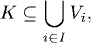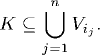# DIFFUSION

A diffusion or a diffusion process is a solution to a stochastic differential equation. In this chapter, we will present some results about diffusion, in particular, Itô diffusion.

# 39.1 Basic Concepts and Facts

Definition 39.1 (Compact Set). A set KR is called a compact set if every open cover of K contains a finite subcover; that is, if {Vi}iI is a collection of open sets such thatthen there exists a finite subcollection {Vij}j=1,2,…,n such thatDefinition 39.2 (Closure). Let ER. The closure of the set E, written as, is the smallest closed set in R that contains E.

Definition 39.3 (Support). The support of a function f on R is the closure of the setDefinition 39.4 (C1,2-Function). A function f on R × R is called a C1,2-function if f(t, x) is continuously differentiable on t (i.e.,is continuous) and twice continuously differentiable on x (i.e., is continuous). ...

Get Measure, Probability, and Mathematical Finance: A Problem-Oriented Approach now with the O’Reilly learning platform.

O’Reilly members experience live online training, plus books, videos, and digital content from nearly 200 publishers.